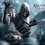# the side length

I have come across a problem two days ago which I could not solve.It's a basic problem but i need a procedure for the problem.

A triangle of sides of lengths a,b,c and the angles opposite to the sides are A,B,C respectively , satisfies the following condition :

a + b = tan (C/2) [ atan (B) + btan (A) ]

The perimeter of the triangle is 36 cm...and the side length c = 16 cm...then the area of triangle is ____ .

[WHEN I ATTEMPTED A QUESTION IN I.M.O. ,THEY SAID IT IS ACTUALLY AN ISOSCELES TRIANGLE.......BUT I DON'T KNOW THE PROOF. ]Note by Vaibhav Reddy
6 years, 7 months ago

This discussion board is a place to discuss our Daily Challenges and the math and science related to those challenges. Explanations are more than just a solution — they should explain the steps and thinking strategies that you used to obtain the solution. Comments should further the discussion of math and science.

When posting on Brilliant:

• Use the emojis to react to an explanation, whether you're congratulating a job well done , or just really confused .
• Ask specific questions about the challenge or the steps in somebody's explanation. Well-posed questions can add a lot to the discussion, but posting "I don't understand!" doesn't help anyone.
• Try to contribute something new to the discussion, whether it is an extension, generalization or other idea related to the challenge.

MarkdownAppears as
*italics* or _italics_ italics
**bold** or __bold__ bold
- bulleted- list
• bulleted
• list
1. numbered2. list
1. numbered
2. list
Note: you must add a full line of space before and after lists for them to show up correctly
paragraph 1paragraph 2

paragraph 1

paragraph 2

[example link](https://brilliant.org)example link
> This is a quote
This is a quote
    # I indented these lines
# 4 spaces, and now they show
# up as a code block.

print "hello world"
# I indented these lines
# 4 spaces, and now they show
# up as a code block.

print "hello world"
MathAppears as
Remember to wrap math in $$ ... $$ or $ ... $ to ensure proper formatting.
2 \times 3 $2 \times 3$
2^{34} $2^{34}$
a_{i-1} $a_{i-1}$
\frac{2}{3} $\frac{2}{3}$
\sqrt{2} $\sqrt{2}$
\sum_{i=1}^3 $\sum_{i=1}^3$
\sin \theta $\sin \theta$
\boxed{123} $\boxed{123}$

Sort by:

You can format your equation by putting \ [ on the left and \ ] on the right (without the spaces).

- 6 years, 7 months ago

you could understand the question right :) now did you solve the problem

- 6 years, 6 months ago

I haven't tried it yet. The problem as it is might be understandable, but if you typeset your problem, it looks better and thus users are way more likely to try the problem. :)

- 6 years, 6 months ago

Let a; b; c be the lengths of the sides of a triangle, and A; B;C, respectively, the angles opposite these sides. Prove that if a + b = tan C/2(a tan B + b tan A); the triangle is isosceles. SORRY,my bad......the upper one is the original question...it appeared in 1966 I.M.O. Now is it easy to solve???

- 6 years, 6 months ago

I see you on every discussion, whether it be a answer to a question or your own question.

- 6 years, 7 months ago